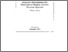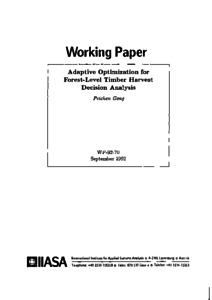# Adaptive Optimization for Forest-Level Timber Harvest Decision Analysis

Gong, P. (1992). Adaptive Optimization for Forest-Level Timber Harvest Decision Analysis. IIASA Working Paper. IIASA, Laxenburg, Austria: WP-92-070Preview
Text
WP-92-070.pdf

## Abstract

This paper describes a method for optimizing multistand timber harvest decisions under uncertainty. The optimal decision policy is approximated by a timber supply function. The supply function is formulated analytically and the supply function coefficients are optimized numerically by maximizing the expected present value of the forest. This method is implemented to the problem of timber harvest decision making under timber price uncertainty for a simple forest with one forest-level activity, i.e., investment in timber harvest capacity, incorporated. Stochastic quasigradient methods are introduced and suggested to be used to optimize the supply function coefficients. Advantages of this method lie in its computational efficiency, flexibility of model formulation, and its application potentials. A numerical example is used to illustrate the application of this method. Sensitivity analysis shows that the level of timber price uncertainty affects both the optimal harvest decision policy and the expected present value of the forest. However, the effects of correlations between timber prices in successive periods are not obvious, probably because of the relatively long decision intervals used in this study. Comparison of the numerical results with the optimal solution to a corresponding deterministic linear program shows that substantially higher expected present value of the forest (12.5-88.4% higher than the linear programming solution, depending on the variance and correlation of the timber price process) can be obtained using this method with the currently formulated timber supply function structure.

Item Type: Monograph (IIASA Working Paper) Adaption and Optimization (ADO) IIASA Import 15 Jan 2016 02:01 27 Aug 2021 17:14 http://pure.iiasa.ac.at/3629View Item